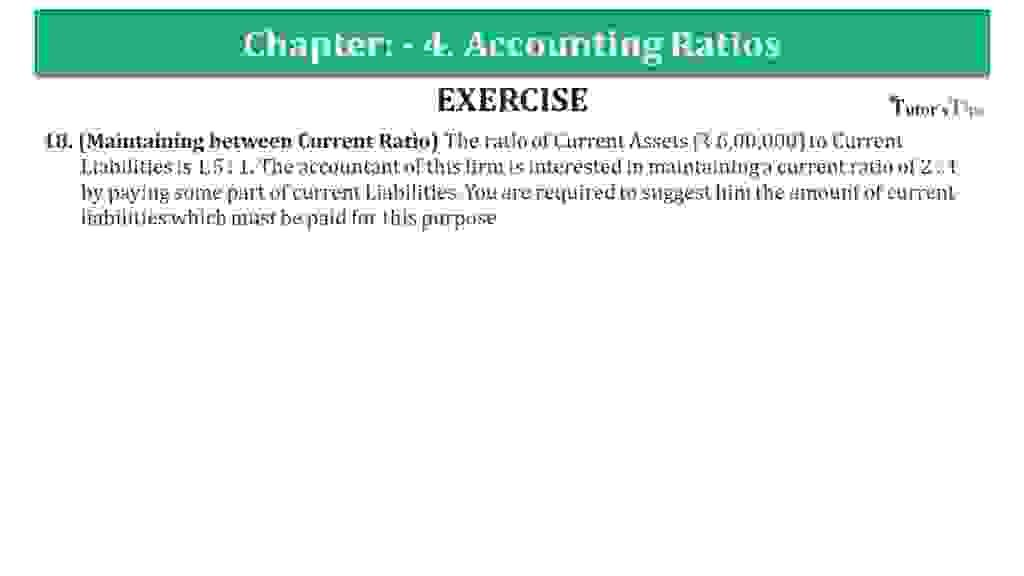# Question 18 Chapter 4 of +2-B – USHA Publication 12 ClassQuestion 18 Chapter 4 of +2-B

I. Liquidity Ratios

18. (Maintaining between Current Ratio) The ratio of Current Assets (₹ 6,00,000) to Current
Liabilities is 1.5: 1. The accountant of this firm is interested in maintaining a current ratio of 2: 1 by paying some part of the Current Liabilities. You are required to suggest to him the number of current liabilities which must be paid for this purpose.

## The solution of Question 18 Chapter 4 of +2-B: –

 Current Assets = ₹ 2,00,000 = 1.5 : 1
 Current Liabilities = ₹ 6,00,000 X 1 1.5
 Let assume Current Liabilities paid = ₹ x New Current Assets = ₹ 6,00,000 – x New Current Liabilities = ₹ 4,00,000 – x
 New Current Ratio = Current Assets Current Liabilities
 2 : 1 = ₹ 6,00,000 – x ₹ 4,00,000 – x ₹ 6,00,000 – x = (₹ 4,00,000 –x) x 2 ₹ 6,00,000 – x = ₹ 8,00,000 – 2x 2x – x = ₹ 8,00,000 – ₹ 6,00,000 x = ₹ 2,00,000 Current Liabilities = ₹ 2,00,000

What are Liquidity Ratios – Formulas and Examples

Comment if you have any question.

Also, Check out the solved question of previous Chapters: –

## Usha Publication – Accountancy PSEB (Class 12) – Volume II – SolutionT.S. Grewal’s Analysis of Financial Statements